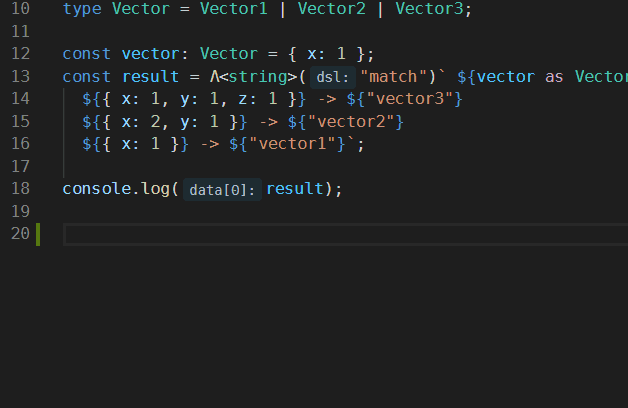# draft-dsl-match

pattern match DSL for typedraft

## Usage no npm install needed!

``````<script type="module">
import draftDslMatch from 'https://cdn.skypack.dev/draft-dsl-match';
</script>``````

# DSL Match ·DSL Match is used in typedraft to support pattern match.

## Inspirations

This DSL is inspired by ts-pattern and tc39/proposal-pattern-matching. Examples and test cases are adapted from ts-pattern.

## Installation

Suppose that you have `typedraft` installed, then:

``````npm i -D draft-dsl-match
``````

and add it to `typedraft.config.ts`:

``````import { PatternMatch } from "draft-dsl-match";

export default {
DSLs: [{ name: "match", dsl: () => new PatternMatch() }],
};
``````

You can use dsl-match-demo as template project to get started quickly.

## Examples

### Data Structure

Support objects, arrays, tuples, Sets, Maps, and all primitive types.

#### Object

``````import { MatchDSL } from "draft-dsl-match";

type Vector1 = { x: number };
type Vector2 = { x: number; y: number };
type Vector3 = {
x: number;
y: number;
z: number;
};
type Vector = Vector1 | Vector2 | Vector3;

const vector: Vector = { x: 1 };
const result = Λ<string>("match")` \${vector as Vector}
\${{ x: 1, y: 1, z: 1 }} -> \${"vector3"}
\${{ x: 2, y: 1 }} -> \${"vector2"}
\${{ x: 1 }} -> \${"vector1"}
`;

console.log(result); // "vector1"
``````

In this example, `import { MatchDSL } from "draft-dsl-match"` is necessary even `MatchDSL` is not used because `Λ` will be translated to `MatchDSL`.

`Λ` is unicode U+039B, it resembles caret and is used to introduce a new context where you can apply a DSL, and `<string>` is used to assert the output type.

"match" is the name of the DSL. You can customize it in `typedraft.config.ts`.

The syntax is straightforward, you put whatever you want to match in the first `\${}`, and the `\${}` at the left of `->` is pattern you want to match, the `\${}` at the right is handler, putting values here implies `() => { return <values>; }`

It's hard to type `Λ`, thus we recommend that you can create code snippet in vscode so that you can type it in this way:The snippet you may want to reuse:

``````{
"dsl": {
"scope": "javascript,typescript,typescriptreact",
"prefix": "dsl",
"body": ["Λ('\$1')` \$2`"],
"description": "use dsl in typedraft"
}
}
``````

#### Array

``````import { MatchDSL, String, __ } from "draft-dsl-match";

type Input = { title: string; content: string }[];

let input: Input = [
{ title: "Hello world!", content: "I‘m a very interesting content" },
{ title: "Bonjour!", content: "I‘m a very interesting content too" },
];

const result = Λ("match")` \${input}
\${[{ title: String, content: String }]} -> \${"a list of posts!"}
\${__} -> \${"something else"}
`;

console.log(result); // "a list of posts!"
``````

`__` will match any value, and `String` is used to denote the `String` type.

#### Tuple

``````import { MatchDSL, Number } from "draft-dsl-match";

const sum = (args: number[]): number => Λ<number>("match")` \${args}
\${[]} -> \${0}
\${[Number, Number]} -> \${([x, y]) => x + y}
\${[Number, Number, Number]} -> \${([x, y, z]) => x + y + z}
\${[Number, Number, Number, Number]} -> \${([x, y, z, w]) => x + y + z + w}
`;

const result = sum([2, 3, 2, 4]);
console.log(result); // 11
``````

``````import { MatchDSL, Number, String, __ } from "draft-dsl-match";

type ArgsType = [string, string] | [number, number] | [any, any];
type RetrunType = number | string | any;

const add = (args: ArgsType): RetrunType => Λ<RetrunType>("match")` \${args as ArgsType}
\${[String, String]} -> \${args => args.join(" ")}
\${[Number, Number]} -> \${([x, y]) => x + y}
\${[__, __]} -> \${args => `the sum is args.join("-"): \${args.join("-")}`}
`;

// hello world
// 3
// the sum is args.join("-"): hi-1
``````

#### Sets and Maps

``````import { MatchDSL, __ } from "draft-dsl-match";

const ContainsGabAndYo = (set: Set<string | number>) => Λ<[boolean, boolean]>("match")` \${set}
\${new Set(["gab", "yo"])} -> \${[true, true]}
\${new Set(["gab"])} -> \${[true, false]}
\${new Set(["yo"])} -> \${[false, true]}
\${__} -> \${[false, false]}
`;

console.log(ContainsGabAndYo(new Set(["gab", "yo", "hello"])));
console.log(ContainsGabAndYo(new Set(["gab", "hello"])));
console.log(ContainsGabAndYo(new Set(["yo", "hello"])));
console.log(ContainsGabAndYo(new Set(["hello"])));
console.log(ContainsGabAndYo(new Set([])));
console.log(ContainsGabAndYo(new Set()));

// [ true, true ]
// [ true, false ]
// [ false, true ]
// [ false, false ]
// [ false, false ]
// [ false, false ]
``````
``````import { MatchDSL, String, __ } from "draft-dsl-match";

const users_map = new Map([
["gab", { name: "gabriel" }],
["angégé", { name: "angéline" }],
]);

const user_pattern = { name: String };

const result = Λ("match")` \${users_map as Map<string, { name: string }>}
\${new Map([
["angégé", user_pattern],
["gab", user_pattern],
])} -> \${map => ({ name: map.get("angégé")!.name + " " + map.get("gab")!.name })}
\${new Map([["angégé", user_pattern]])} -> \${map => map.get("angégé")}
\${new Map([["gab", user_pattern]])} -> \${map => map.get("gab")}
\${__} -> \${{ name: "unknown" }}
`;

console.log(result); // { name: 'angéline gabriel' }
``````

### Predicate

Predicate can also be used at the left side of `->`:

``````import { MatchDSL, __ } from "draft-dsl-match";

const values = [
{ value: 1, expected: false },
{ value: -2, expected: false },
{ value: 3, expected: false },
{ value: 100, expected: false },
{ value: 20, expected: true },
{ value: 39, expected: true },
];

values.forEach(({ value, expected }) => {
const result = Λ<boolean>("match")` \${value}
\${(x: number) => x > 10 && x < 50} -> \${true}
\${__} -> \${false}
`;
console.log(result === expected);
});

// true
// true
// true
// true
// true
// true
``````

### Properties selection

You can use matched value in this way:

``````import { MatchDSL, __, use } from "draft-dsl-match";

const y = Λ("match")` \${["get", 2]}
\${["get", use("y")]} -> \${(_, { y }) => y}
`;

console.log(y); // 2
``````

The second param of handler is an object that contains values you want to "use".